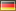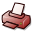# The ABC Effect in Double-Pionic Fusion to Deuterium

## DSpace Repository

 Dateien:

 URI: Dokumentart: Dissertation Date: 2009 Language: English Faculty: 7 Mathematisch-Naturwissenschaftliche Fakultät Department: Physik Advisor: Clement, Heinz (Prof. Dr. ), Wagner, Gerhard (Prof. Dr.) Day of Oral Examination: 2009-04-17 DDC Classifikation: 530 - Physics Keywords: Masse Other Keywords:ABC Effekt , Überhöhung , kleine invariante Masse von 2 Pionen , WASA Detektor , CELSIUS Speicherring , Uppsala/SchwedenABC Effect , Low-mass enhancement , Invariant mass of two pions , WASA Detector , CELSIUS ring , Uppsala/Sweden License: Order a printed copy:Print-on-Demand Show full item record

### Inhaltszusammenfassung:

Der ABC-Effekt wird bei der 2$\pi$-Produktion in Nukleon-Nukleon oder Nukleon-Kern-Stößen beobachtet, wenn eine Fusion des Projektils mit Targetnukleonen auftritt. Als ABC-Effekt bezeichnet man eine Überhöhung des differentiellen Wirkungsquerschnitts bei kleinen invarianten $\pi\pi$-Massen. Er wurde zuerst in inklusiven Messungen der doppelt-pionischen Fusion von Deuterium und Wasserstoff zu $^3He$ entdeckt. Später wurde er auch in inklusiven Messungen der Reaktion $np \to d\pi\pi$ mit einem Neutronen-Strahl beobachtet. In dieser Arbeit werden erste exklusive Messungen der Reaktion $pd \to pd\pi^0\pi^0$ durchgeführt und zwar bei Strahlenergien von $T_p =$ 1.03 und 1.35 GeV am CELSIUS Speicherring in Uppsala/Schweden. Der WASA Detektor kann geladene und neutrale Teilchen im nahezu gesamten 4$\pi$-Raumwinkelbereich nachweisen. Deuteronen wurden im {\it Forward Detector} gemessen, während die 4 Photonen, die aus dem Zerfall von 2$\pi^0$ entstanden, im {\it Central Detector} nachgewiesen wurden. Die gewählten Strahlenergien sind sehr nahe am Maximum des totalen Wirkungsquerschnittes der Reaktion $pn \to d\pi^+\pi^-$, der bei den vorherigen inklusiven Messungen erhalten wurde. Deswegen sind sie optimal für die Untersuchung des ABC-Effektes geeignet. Die Reaktion $pn \to d\pi^0\pi^0$ wurde als quasifreie Reaktion $pd \to p_{spec}d\pi^0\pi^0$ mit einem Spektator-Proton $p_{spec}$ sehr kleiner Energie gemessen. Weil alle Teilchen außer dem Spektator-Proton gemessen wurden, konnte sein Impuls mit Hilfe eines dreifach überbestimmten kinematischen Fits rekonstruiert werden. Mithin konnte die Fermi-Bewegung im Deuteron-Target genutzt werden, um bei gegebener Strahlenergie einen Bereich Relativenergien im $pn$-System zu überdecken. Der $\pi^0\pi^0$-Kanal ist rein isoskalar und frei von Isovektor-Beiträgen. Er zeigt eine große Überhöhung im $M_{\pi^0\pi^0}$-Spektrum an der $\pi\pi$-Schwelle, die viel größer ist als in vorherigen inklusiven Messungen beobachtet und mit $\Delta\Delta$-Rechnungen vorhergesagt wurde. Im Gegensatz zu letzteren wie auch zu den inklusiv gemessenen Daten wurde eine Überhöhung bei hohen invarianten Massen $M_{\pi^0\pi^0}$ nicht beobachtet und inzwischen als 3$\pi$ und $\eta$-Meson Produktion interpretiert. Da die verfügbaren $\Delta\Delta$-Modelle die exklusiv gemessenen Daten nicht beschreiben können, wird hier eine andere mögliche Erklärung vorgestellt. Die Überhöhung bei niedrigen invarianten Massen steht in einem engen Zusammenhang mit der beobachteten resonanzartigen Struktur des totalen Wirkungsquerschnittes. Die exklusiv gemessenen Daten können beschrieben werden, wenn man eine Resonanz im isoskalaren $pn$-System annimmt, die überwiegend über ein isoskalares $\Delta\Delta$-System zerfällt. Mit diesem so genannten s-Kanal-Resonanz-Ansatz erhält man eine sehr gute Beschreibung des totalen Wirkungsquerschnitts sowie der differentialen Spektren. Masse und Breite dieser isoskalaren dibaryonischen Resonanz ergeben sich zu $M_R \approx$ 2.36 GeV/c$^2$ und $\Gamma_R \approx$ 80 MeV. Diese verblüffenden Resultate wurden bereits in Phys. Rev. Lett. {\bf 102}, 052301 (2009) publiziert.

### Abstract:

The ABC effect is observed in the 2$\pi$-production in nucleon-nucleon or nucleon-nucleus collisions, if fusion of the projectile with the target nucleons occurs. The ABC effect is a low-mass enhancement in the $\pi\pi$ invariant mass spectrum. It was first discovered in the inclusively measured double pionic fusion of deuterons and protons to $^3$$He$. Lateron it has also been observed in inclusive measurements of the reaction $np \to d\pi\pi$ with a neutron beam. In this work the first exclusive measurements of the reaction $pd \to pd\pi^0\pi^0$ have been carried out at beam energies of $T_p =$ 1.03 and 1.35 GeV at CELSIUS storage ring in Uppsala/Sweden. The WASA detector with almost full 4$\pi$ solid angle coverage allows to detect charged and neutral particles. Deuterons were measured in the {\it Forward Detector}, whereas the 4 photons, which originated from 2$\pi^0$ decay, detected in the {\it Central Detector}. The selected energies are close to the maximum cross section of the reaction $pn \to d\pi^+\pi^-$ observed in the previous inclusive measurements. Therefore they are optimal for the study of the ABC effect. The reaction $pn \to d\pi^0\pi^0$ has been measured as quasifree $pd \to p_{spec}d\pi^0\pi^0$ reaction with a spectator proton $p_{spec}$ of very small momentum. Since all particles except of the spectator proton have been measured, the spectator 4-momentum could be reconstructed by kinematical fits with 3 overconstraints. Hence one could exploit the Fermi motion of the target neutron to cover a range of relative energies in the $pn$-system for a given beam energy. The $\pi^0\pi^0$ channel, which is purely isoscalar and free of any isovector contributions, shows a large low-mass enhancement in the $M_{\pi^0\pi^0}$ spectrum, which is much larger than observed in the inclusive measurements and also larger than predicted in previous $\Delta\Delta$ calculations. In contrast to these and also to the inclusive data a high-mass enhancement in the $M_{\pi^0\pi^0}$ spectrum was not observed and is meanwhile interpreted as 3$\pi$ and $\eta$-meson production. Since the available $\Delta\Delta$ calculations are not successful in the description of the exclusively measured data, another explanation is presented here. The enhancement in the low invariant mass $M_{\pi^0\pi^0}$ is strongly correlated with the observed resonance-like structure in the total cross section. Indeed, all exclusive data can be described, if one assumes a resonance in the isoscalar $pn$-system, which dominantly decays via the isoscalar $\Delta\Delta$ system. With this so-called s-channel resonance ansatz a very good description of the data in the total cross section as well as in the differential spectra has been achieved. Mass and width of this isoscalar dibaryonic resonance are $M_R \approx$ 2.36 GeV/c$^2$ and $\Gamma_R \approx$ 80 MeV, respectively. These intriguing results have already been published in Phys. Rev. Lett. {\bf 102}, 052301 (2009).